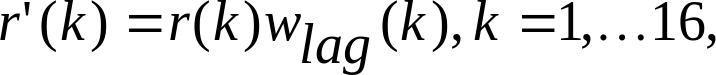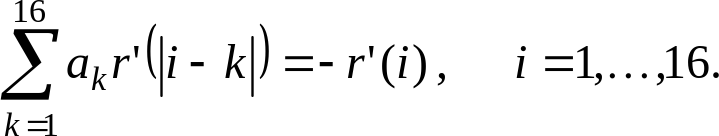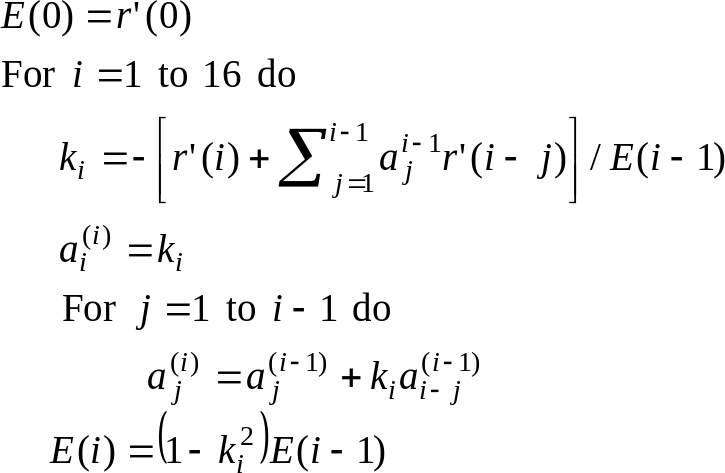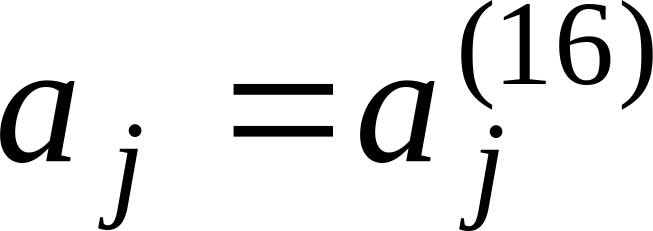## 5.2.2 Levinson‑Durbin algorithm

26.1903GPPAdaptive Multi-Rate - Wideband (AMR-WB) speech codecSpeech codec speech processing functionsTranscoding functionsTS

The modified autocorrelationsandare used to obtain the LP filter coefficients ak,k=1,…,16 by solving the set of equations.( 9 )

The set of equations in (9) is solved using the Levinson-Durbin algorithm . This algorithm uses the following recursion:The final solution is given as,j=1,…,16.

The LP filter coefficients are converted to the ISP representation  for quantization and interpolation purposes. The conversions to the ISP domain and back to the LP filter domain are described in the next two sections.